Here we are providing Class 11 chemistry Important Extra Questions and Answers Chapter 10 The s-Block Elements. Chemistry Class 11 Important Questions are the best resource for students which helps in Class 11 board exams.

## Class 11 Chemistry Chapter 10 Important Extra Questions The s-Block Elements

### The s-Block Elements Important Extra Questions Very Short Answer Type

Question 1
Which element is found in chlorophyll?
Magnesium.

Question 2.
Name the elements (alkali metals) which form superoxide when heated in excess of air.
Potassium, rubidium and caesium.

Question 3.
Why is the oxidation state of Na and K always + 1?
It is due to their high second ionisation enthalpy and stability of their ions [Na+, K+].

Question 4.
Name the metal which floats on the water without any apparent reaction with water.
Lithium floats on the water without any apparent reaction to it.

Lithium Oxide Formula. Lithium oxide also lithia is an inorganic compound.

Question 5.
Why do group 1 elements have the lowest ionisation enthalpy?
Because of the largest size in their respective periods, solitary electron present in the valence shell can be removed by supplying a small amount of energy.

Question 6.
Why does the following reaction
C – Cl + MF → C – F + MCI
proceed better with KF than with NaF?
Because larger K+ cation stabilises larger anion.

Question 7.
Amongst Li, Na, K, Rb, Cs, Fr which one has the highest and which one has the lowest ionisation enthalpy?
Li has the highest and Fr has the lowest ionisation enthalpy.

Question 8.
What is the general electronic configuration of alkali metals in their outermost shells?
ns1 where n = 2 to 7.

Question 9.
What is meant by dead burnt plaster?
It is anhydrous calcium sulphate (CaS04).

Question 10.
Name three forms of calcium carbonate.
Limestone, chalk, marble.

Question 11.
Which member of the alkaline earth metals family has
(i) least reactivity
Be

(ii) lowest density
Ca

(iii) highest boiling point
Be

(iv) maximum reduction potential?
Be.

Question 12.
Why does lithium show anomalous behaviour?
Due to its small size and high charge/size ratio.

Question 13.
Out of LiOH, NaOH, KOH which is the strongest base?
KOH.

Question 14.
Write the balanced equations for the reaction between
(a) Na2O2 and water
2Na2O2 + 2H2O → 4NaOH + O2

(b) KO2 and water
2KO2 + 2H2O → 2KOH + H2O + O2

(c) Na2O and CO2.
Na2O4 + CO2 → Na2CO3

Question 15.
Arrange the following in order of increasing covalent character MCI, MBr. MF, MI (where M = alkali metal]
MF < MCI < MBr < Ml.
With the increasing size of anion, covalent character increases

Question 16.
Name an element that is invariably bivalent and whose oxide is soluble in excess of NaOH and its dipositive ion has a noble gas core.
The element is beryllium (Be) which forms a divalent ion and has a noble gas core [He] 2s2
Be2+ = 1 s2
BeO + 2NaOH → Na2BeO2 + H2O

Question 17.
Arrange the following in the increasing order of solubility in water:
MgCl2, CaCl2, SrCl2, BaCl2.
BaCl2 < SrCl2 < CaCl2 < MgCl2.

Question 18.
State the reason for the high solubility of beryllium chloride in organic solvents.
Beryllium chloride is a covalent compound.

Question 19.
Which alkali carbonate decomposes on heating to liberate CO2?
Lithium carbonate [Li2CO3]Question 20.
Why is the solution of an alkali metal in ammonia blue?
Due to the presence of ammoniated electrons.

Question 21.
Beryllium oxide has a high melting point. Why?
Due to its polymeric nature.

Question 22.
Mention the chief reasons for the resemblance between beryllium and aluminium.
Both Be2+ and Al3+ ions have high polarising power.

Question 23.
State any one reason for alkaline earth metals, in general, having a greater tendency to form complexes than alkali metals.
Because of their small size and high charge, alkaline earth metals have a tendency to form complexes.

Question 24.
Amongst alkali metals why is lithium regarded as the most powerful reducing agent in aqueous solution?
Lithium is the best reducing agent because it has the lowest reduction potential:
Li+ + e → Li(s) ERed = – 3.07V.

Question 25.
Name the metal amongst alkaline earth metals whose salt does not impart any colour to a non-luminous flame.
Beryllium does not impart colour to a non-luminous flame.

Question 26.
What is the difference between baking soda and baking powder?
Baking soda is sodium bicarbonate (NaHCO3) while baking powder is a mixture of sodium bicarbonate fNaHC03) and Potassium hydrogen tartrate.

Question 27.
Why does table salt get wet in the rainy season?
Table salt contains impurities of CaCl2 and MgCl which being deliquescent compounds absorb moisture from the air during the rainy season.

Question 28.
Sodium readily forms Na+ ion, but never Na2+ ion. Explain.
After the removal of 1 electron from Na, it has been left with a noble gas core [Ne] which is closer to the nucleus and requires more energy.

Question 29.
Which compound of sodium is used
(i) as a component of baking powder
NaHCO3

(ii) for softening hard water.
Na2CO3.

Question 30.
Anhydrous calcium sulphate cannot be used as Plaster of Paris. Give reason.
Because it does not have the ability to set like plaster of Paris.

Question 31.
Which of the following halides is insoluble in water?
CaF2, CaCl2, CaBr2 and Cal2.
CaF2.

Question 32.
Predict giving reason the outcome of the reaction.
Lil + KF → …………
LiI + KF → LiF + KI
Larger cation stabilises larger anion.

Question 33.
Name three metal ions that play important role in performing several biological functions in the animal body.
Na+, K+, Ca2+, Mg2+, etc.

Question 34.
Calcium metal is used to remove traces of air from vacuum tubes. Why?
Calcium has a great affinity for oxygen and nitrogen.

Question 35.
Why is sodium kept in kerosene oil?
To prevent its contact with oxygen and moist air, because sodium reacts with them.

Question 36.
What is brine?
An aqueous solution of NaCl in water.

Question 37.
Why caesium can be used in photoelectric cells while lithium cannot be?
Cs has the lowest & Li highest ionisation enthalpy. Hence Cs can lose electron very easily while lithium cannot.

Question 38.
In an aqueous solution, the Li+ ion has the lowest mobility. Why?
Li+ ions are highly hydrated in an aqueous solution.

Question 39.
Lithium has the highest ionisation enthalpy in group-1, yet it is the strongest reducing agent. Why?
This is because Li has the highest oxidation potential.
Li → Li+ + e
ox = + 3.07

Question 40.
Name one reagent or one operation to distinguish between
(i) BeSO4 and BaSO4
BeSO4 is soluble in water while BaSO4 is not.

(ii) Be(OH)2 and Ba(OH)2
Be(OH)2 dissolves in alkali, while Ba(OH)2 does not.

Question 41.
Which alkaline earth metal hydroxide is amphoteric?
Be(OH)2.

Question 42.
Francium (Fr).

Question 43.
Which alkaline earth metal is radioactive?

Question 44.
Name the alkali metal which shows a diagonal relationship with Mg.
Lithium (Li).

Question 45.
Give the chemical formula of carnallite.
KCl.MgCl2.6H2O.

Question 46.
Arrange CaSO4, SrSO4 & BaSO4 in order of decreasing solubility.
The order of decreasing solubility of these sulphates is CaSO4, SrSO4 & BaSO4.

Question 47.
Why is K more reactive than Na?
Since the valence electron of K is relatively at a greater distance than Na & it requires lesser energy to remove it.

Question 48.
Why does Beryllium show similarities with Al?
Because of their similarity in charge/radius ratio (Be2+ = 0.064 & Al3+ = 0.660)

Question 49.
What is magnesia?
Magnesium oxide (MgO).

Question 50.
How is Potassium extracted?
By electrolysis of a fused solution of KOH.

### The s-Block Elements Important Extra Questions Short Answer Type

Question 1.
Why the solubility of alkaline metal hydroxides increases down the group?
If the anion and the cation are of comparable size, the cationic radius ‘vill influence the lattice energy. Since lattice energy decreases much more than the hydration energy with increasing ionic size, solubility will increases as we go down the group. This is the case of alkaline earth metal hydroxides.

Question 2.
Why the solubility of alkaline earth metal carbonates and sulphates decreases down the group?
If the anion is large compared to the cation, the lattice; energy will remain almost constant within a particular group. Since the hydration energies decrease down the group, solubility will decrease as found for alkaline earth metal carbonates and sulphates.

Question 3.
Why cannot potassium carbonate be prepared by the SOLVAY process?
Potassium carbonate cannot be prepared by the SOLVAY process because potassium bicarbonate (KHCO3) is highly soluble in water, unlike NaHCO3 which was separated as crystals. Due to its high solubility KHCO3 cannot be precipitated by the addition of ammonium bicarbonate to a saturated solution of KCl.

Question 4.
What are the main uses of calcium and magnesium?
Main uses of calcium:

1. Calcium is used in the extraction of metals from oxides which are difficult to reduce with carbon.
2. Calcium, due to its affinity for O2 and N2 at elevated temperatures, has often been used to remove air from vacuum tubes.

Main uses of Magnesium:

1. Magnesium forms alloys with Al, Zn, Mn and Sn. Mg-Al alloys being light in mass are used in aircraft construction.
2. Magnesium (powder and ribbon) is used in flashbulbs, powders incendiary bombs and signals.
3. A suspension of Mg(OH)2 in water is used as an antacid in medicine.
4. Magnesium carbonate is an ingredient of toothpaste.

Question 5.
What is meant by the diagonal relationship in the periodic table? What is it due to?
It has been observed that some elements of the second period show similarities with the elements of the third period situated diagonally to each other, though belonging to different groups. This is called a diagonal relationship.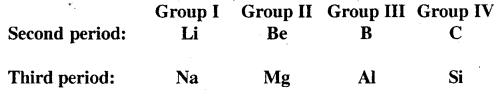The cause of the diagonal relationship is due to the similarities in properties such as electronegativity, ionisation energy, size etc. between the diagonal elements. For example on moving from left to right across a period, the electronegativity increases, which on moving down a group, electronegativity decreases. Therefore on moving diagonally, two opposing tendencies almost cancel out and the electronegativity values remain almost the same as we move diagonally.

Question 6.
Why is the density of potassium less than that of sodium?
Generally, in a group density increases with the atomic number, but potassium is an exception. It is due to the reason that the atomic volume of K is nearly twice Na, but its mass (39) is not exactly double of Na (23). Thus the density of potassium is less than that of sodium.

Question 7.
The hydroxides and carbonates of sodium and potassium are easily soluble in water while the corresponding salts of magnesium and calcium are sparingly soluble in water. Explain.
Sodium and potassium ions (Na+ and K+) are larger than the corresponding Mg2+ and Ca2+ ions. Due to this lattice energy of Mg(OH)2, Ca(OH)2, MgCO3 and CaCO3. (Lattice energy is defined as the energy required to convert one mole of the ionic lattice into gaseous ions.

Thus lattices with smaller ions have higher lattice energies). The hydration energies of Mg2+ and Ca2+ are higher than Na+ and K+ because of their smaller sizes. But the difference in lattice energies is much more. Therefore, the hydroxides and carbonates of Mg2+ and Ca2+ are insoluble in water because of their higher lattice energies.

Question 8.
Why is it that the s-block elements never occur in free state/nature? What are their usual modes of occurrence and how are they generally prepared?
The elements belonging to the s-block in the periodic table (i.e. alkali and alkaline earth metals) are highly reactive because of their low ionisation energy. They are highly electropositive forming positive ions. So they are never found in a free state.

They are widely distributed in nature in the combined state. They occur in the earth’s crust in the form of oxides, chlorides, silicates and carbonates.

Generally, a group I metals are prepared by the electrolysis of fused solution.
For example:

1.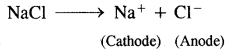At cathode: Na+ + e → Na
At anode: Cl → Cl + e
Cl + Cl → Cl2

2. KOH ⇌ K+ + OH
At cathode: K+ + e→ K
At anode: 4OH → 4OH + 4e
4OH → 2H2O + O2
or
4OH → 2H2O + O2 + 4e
These metals are highly reactive and therefore cannot be extracted by the usual methods, because they are strong reducing agents.

Question 9.
Explain what happens when
(i) Sodium hydrogen carbonate is heated.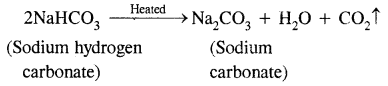(ii) Sodium with mercury reacts with water.
2Na-Hg + 2H2O → 2NaOH + H2 ↑ + 2Hg

(iii) Fused sodium metal reacts with ammonia.Question 10.
What is the effect of heat on the following compounds?
(a) Calcium carbonate(b) Magnesium chloride hexahydrate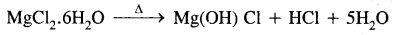(c) Gypsum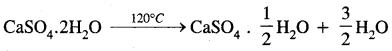(d) Magnesium sulphate heptahydrate.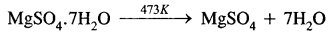Question 11.
Why is it that the s-block elements never occur in a free state? What are their usual modes of occurrence?
The elements belonging to s-block in the periodic table. These metals (Alkali & alkaline earth metals) are highly reactive because of their low ionization energy. They are highly electropositive forming positive ions. So they are never found in a free state. They are widely distributed in nature in a combined state. They occur in the earth’s crust in the form of oxides, chlorides, silicates & carbonates.

Question 12.
Explain what happens when:
(a) Sodium hydrogen carbonate is heated.
Sodium hydrogen carbonate on heating decomposes to sodium carbonate.(b) Sodium with Mercury reacts with water.
When sodium with mercury reacts with water. It produces sodium hydroxide.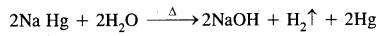Question 13.
What is the effect of heat on the following compounds:
(a) Calcium carbonate(b) Magnesium chloride hexahydrate(c) Gypsum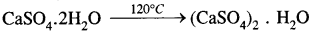Question 14.
State as to why
(a) An aqueous solution of sodium carbonate gives an alkaline test.
Sodium carbonate gets hydrolise by water to form an alkaline solution.
CO32- + H2O → HCO3 + OH
Due to this, it gives an alkaline test.

(b) Sodium is prepared by electrolytic method & not by chemical method.
Sodium is a very strong reducing agent. Therefore, it cannot be isolated by a general reduction of its oxides or other compounds. The metal formed by electrolysis will immediately react with water forming hydroxides. So, sodium is prepared by the electrolytic method only.

(c) Lithium on being heated in the air mainly forms mono-oxide & not the peroxides.
Lithium is the least reactive but the strongest reducing agent of all the alkali metals. It combines with air, it forms mono-oxide only because it does not react with excess air.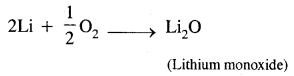Question 15.
Like Lithium in group-I, beryllium shows anomalous behaviour in group II. Write three such properties of beryllium which makes it anomalous in the group.
1. Beryllium has an exceptionally small atomic size, due to high ionization energy & small atomic size it forms compounds that are largely covalent & its salts are hydrolysed easily.

2. Beryllium does not exhibit coordination N. more than four as in its valence shell (n = 2). There are only four orbitals. The remaining member of the group has co-ordination no. six by making use of group have co-ordination no. six by making use of some d-orbitals.

3. The oxides & hydroxides of beryllium quite unlike the other elements, in this group are amphoteric in nature.

Question 16.
Explain which one Na or K has a larger atomic radius?
Potassium has a larger atomic size than sodium because, in K, the outermost electron is in the fourth energy state (4s1) while in Na, the outer-most electron is in the third energy state (3s1). That is r (K) > r (Na) since the fourth energy state (n = 4) in K is farther away from the nucleus than the third energy state (n = 3) in Na.

Question 17.
The alkali metals (group-1 elements) form only unipositive cations (M+) & not bivalent cations (M+2), Give reason.

Alkali metals have an ns1 valence shell configuration. They can give up this electron to form univalent cations & attain stable electronic configuration like their nearest inner gas. Now the removal of an electron from the closed-shell configuration requires a very large amount of energy. Which is not available in chemical reactions. Therefore, the alkali metals form a univalent cation (M+) & No divalent cations (M2+) are formed.

Question 18.
Complete the following equations:
(a) Ca + H2O →?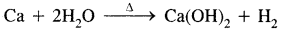(b) Ca(OH)2 + Cl2 →?
2Ca(OH)2 + l → Ca(OCl)2 + CaCl2 + H2O

(c) BeO + NaOH →?
BeO + 2NaOH → Na2BeO2 + H2O

Question 19.
How calcium carbonate can be changed into
(a) Calcium sulphate
(b) Calcium oxide?
Write a balanced chemical equation in each case.
(a) When calcium carbonate is heated with dil. sulphuric acid, calcium sulphate is obtained.
CaCO3(s) + H2SO4(aq) → CaSO4(s) + CO2(g) + H2O(l)

(b) Calcium carbonate is heated at a high temperature between 1070 – 1270 K. It decomposes to give calcium oxide & carbon dioxide.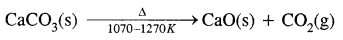Question 20.
Explain the following phenomenon by means of balanced equations:
(a) When exhaling is made through a tube passing into a solution of lime water, the solution becomes turbid.
Ca(OH)2 (l) + CO2 (g) → CaCO3 (s) + H2O (l)

(b) The turbidity of solution in (a) eventually disappears when continued exhaling is made through it.
CaCO3(s) + H2O(l) + CO2(g) → Ca(HCO3)2(aq)

(c) When the solution obtained in (B) is heated turbidity re-appears.
Ca(HCO3)2(aq) → CaCO3(s) + H2O(l) + CO2(g)

Question 21.
Describe the reactivity of alkaline earth metals with water.
The reactivity of alkaline earth metals with water increases with increasing atomic numbers.

1. Beryllium does not react readily even with boiling water.
2. Magnesium reacts very slowly with cold water. While it reacts with hot water at an appreciable rate & liberates hydrogen gas.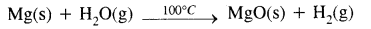3. Calcium, strontium & Barium react vigorously even with cold water & form hydroxides & liberate hydrogen gas
M(s) + H2O(l) → M(OH)2 + H2(g)
[M = Ca, Sr, Ba]

Question 22.
Compare the reactivity of alkali metals with water.
All the alkali metals displace hydrogen from water i.e.
2M + 2H2O → 2MOH + H2

Lithium reacts slowly with water, sodium reacts violently & Cs reacts so vigorously that the reaction if not controlled can lead to an explosion.
2Li(s) + 2H2O(aq) → 2LiOH (aq) + H2(g) Slow
2Na(s) + 2H2O(aq) → 2NaOH (aq) + H2(g) Fast
2Cs(s) + 2H2O(aq) → 2CsOH (aq) + H2(g) Violent

Question 23.
Alkaline earth metals are harder than alkali metals. Give reason.
Alkaline earth metals are harder than alkali metals because:

1. The atomic radius of alkaline metals is small, atomic mass is high & density is larger than those of alkali metals. Alkaline earth metals have closed packed crystal structure.
2. In alkaline earth metals, the metallic bonding in its crystal is very strong as compared to the crystal of an alkali metal. Therefore, the atoms in the crystal of alkaline earth metals are strongly bonded.

Question 24.
Differentiate amongst Quick lime, Lime water & Slaked lime.Question 25.
The mobilities of alkali metal ions in aqueous solution follow the order
Li+ < Na+ < K+ < Rb+ < Cs+ Give reasons.
Smaller ions are expected to have more mobility. But smaller ions such as Li+, Na+ due to their higher charge density tend to undergo hydration. Hydration increases the mass & effective size of the smaller ion & as a result, the mobility of ions show the trend given.

Question 26.
How is sodium peroxide manufactured? What happens when it reacts with chromium (III) hydroxide? Give it’s two uses.
Sodium peroxide is manufactured by heating sodium metal on aluminium trays in the air (free-from CO2).
2Na + O2 (air) → Na2O2

Sodium Peroxide oxidises chromium (III) hydroxide to sodium chromate.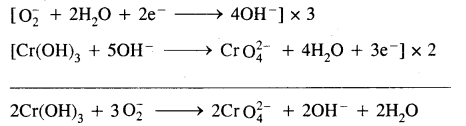Uses:

• It is used as a bleaching agent because of its oxidising, power.
• It is used in the manufacture of dyes & many other chemicals such as benzoyl peroxide, sodium perborate etc.

Question 27.
List three properties of Lithium in which it differs from the rest of the alkali metals.
(a) Lithium is much harder, its melting & boiling points are higher than the other alkali metals.
(b) Lithium is the least reactive but the strongest reducing agent among all the alkali metals.
(c) LiCl is deliquescent & crystallise as a hydrate, LiCl.2H2O whereas other alkali metal chlorides do not form hydrates.

Question 28.
Why is LiF almost insoluble in water whereas LiCl is soluble not only in water but also in acetone?
The low solubility of LiF is due to its high lattice enthalpy. LiCl has much higher solubility in water. This is due to the small size of Li+ ion & much higher hydration energy.

Question 29.
What happens when
(1) Calcium nitrate is heated,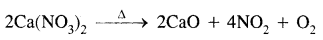(2) Chlorine reacts with slaked lime(3) Quick lime is heated with silica.Question 30.
Arrange the following in order of property mentioned:
(1) Mg(OH)2, Sr(OH)2, Ba(OH)2, Ca(OH)2
(Increasing ionic solubility in water)
Mg(OH)2, Ca(OH)2, Sr(OH)2, Ba(OH)2

(2) BeO, MgO, BaO, CaO
(Increasing basic character)
BeO, MgO, CaO, BaO

(3) BaCl2, MgCl2, BeCl,, CaCl2
(Increasing ionic character)
BeCl2, MgCl2, CaCl2, BaCl2

### The s-Block Elements Important Extra Questions Long Answer Type

Question 1.
What raw materials are used for making cement? Describe the manufacture of Portland cement. What is its approximate composition?
Raw materials: The raw materials required for the manufacture of cement are limestone, stone and clay, limestone in calcium carbonate, CaCO3 and it provides calcium oxide, CaO. Clay- is a hydrated aluminium silicate, Al2O3 2Si02.2H20 and it provides alumina as well as silica. A small amount of gypsum, CaS04.2H20 is also required. It is added in calculated quantity in order to adjust the rate of setting of cement.

Manufacture: Cement is made by strongly heating a mixture of limestone and clay in a rotatory kiln. Limestone and clay are finely powdered and a little water is added to get a thick paste called slurry. The slurry is led into a rotatory kiln from the top through the hopper.

The hot gases produce a temperature of about 1770-1870 K in the kiln. At this high temperature, the limestone and clay present in the slurry combine to form cement in the form of small pieces called clinker. This clinker is mixed with 2 – 3 % by weight of gypsum (CaSO4 .2H2O) to regulate the setting time and is then ground to an exceedingly fine powder.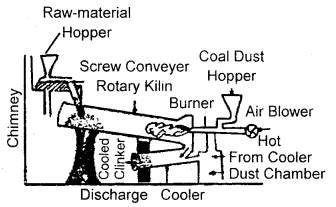Manufacture of cementWhen mixed with water the cement reacts to form a gelatinous mass which sets to a hard mass when three-dimensional cross-links are formed between
…………..Si — O — Si……… and …….. Si — O — Al…… chains.

Composition of cement:
CaO = 50 – 60%
SiO2 = 20 – 25%
Al2O3 = 5 – 10%
MgO = 2 – 3%
Fe2O3 = 1 – 2%
SO3= 1 – 2%

For a good quality cement, the ratio of silica (SiO2) and alumina (Al2O3) should be between 2.5 to 4.0. Similarly, the ratio of lime (CaO) to the total oxide mixtures consisting of SiO2, Al2O3 and Fe2O3 should be roughly 2: 1. If lime is in excess, the cement cracks during setting. On the other hand, if lime is less than required, the cement is weak in strength. Therefore, the proper composition of cement must be maintained to get cement of good quality.